# 4 digit by 1 digit Multiplication

### 4 digit by 1 digit Multiplication Examples

4 digit by 1 digit Multiplication – Example 1

Find the product.

3254 x 5 = ?

Explanation

Step I: Multiply 4 in ones place with 5
4 x 5 = 20
Since, the number is more than 9, we need to carry over 2 to the tens place.
Write 0 in ones place.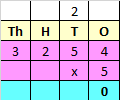Step II: Multiply 5 in tens place with 5
5 x 5 = 25
Add the carry over of 2 to 25
25 + 2 = 27
Since, the number is more than 9, we need to carry over 2 to the hundreds place.
Write 7 in tens place.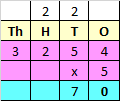Step III: Multiply 2 in hundreds place with 5
2 x 5 = 10
Add the carry over of 2 to 10
10 + 2 = 12
Since, the number is more than 9, we need to carry over 1 to the thousands place.
Write 2 in hundreds place.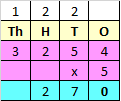Step IV: Multiply 3 in thousands place with 5
3 x 5 = 15
Add the carry over of 1 to 15
15 + 1 = 16
Since, the number is more than 9, we need to carry over 1 to the ten thousands place.
Write 1 in ten thousands place and 6 in thousands place.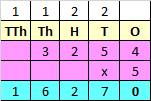Hence, 3254 x 5 = 16270

4 digit by 1 digit Multiplication – Example 2

Find the product.

5234 x 8 = ?

Explanation

Step I: Multiply 4 in ones place with 8
4 x 8 = 32
Since, the number is more than 9, we need to carry over 3 to the tens place.
Write 2 in ones place.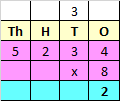Step II: Multiply 3 in tens place with 8
3 x 8 = 24
Add the carry over of 3 to 24
24 + 3 = 27
Since, the number is more than 9, we need to carry over 2 to the hundreds place.
Write 7 in tens place.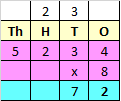Step III: Multiply 2 in hundreds place with 8
2 x 8 = 16
Add the carry over of 2 to 16
16 + 2 = 18
Since, the number is more than 9, we need to carry over 1 to the thousands place.
Write 8 in hundreds place.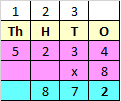Step IV: Multiply 5 in thousands place with 8
5 x 8 = 40
Add the carry over of 1 to 40
40 + 1 = 41
Since, the number is more than 9, we need to carry over 4 to the ten thousands place.
Write 4 in ten thousands place and 1 in thousands place.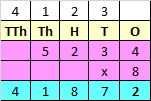Hence, 5234 x 8 = 41872